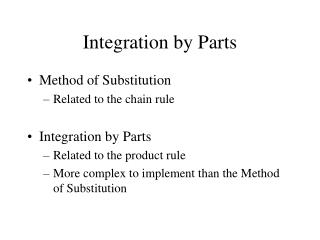DownloadDownload PresentationIntegration by Parts

# Integration by Parts

Download Presentation## Integration by Parts

- - - - - - - - - - - - - - - - - - - - - - - - - - - E N D - - - - - - - - - - - - - - - - - - - - - - - - - - -
##### Presentation Transcript

1. Integration by Parts • Method of Substitution • Related to the chain rule • Integration by Parts • Related to the product rule • More complex to implement than the Method of Substitution

2. Derivation of Integration by Parts Formula Let u and v be differentiable functions of x.

3. Derivation of Integration by Parts Formula Let u and v be differentiable functions of x. (Product Rule)

4. Derivation of Integration by Parts Formula Let u and v be differentiable functions of x. (Product Rule) (Integrate both sides)

5. Derivation of Integration by Parts Formula Let u and v be differentiable functions of x. (Product Rule) (Integrate both sides) (FTC; sum rule)

6. Derivation of Integration by Parts Formula Let u and v be differentiable functions of x. (Product Rule) (Integrate both sides) (FTC; sum rule)

7. Derivation of Integration by Parts Formula Let u and v be differentiable functions of x. (Product Rule) (Integrate both sides) (FTC; sum rule) (Rearrange terms)

8. Integration by Parts Formula • What good does it do us? • We can trade one integral for another. • This is only helpful if the integral we start with is difficult and we can trade it for a good (i.e., solvable) one.

9. Classic Example

10. Helpful Hints • For u, choose a function whose derivative is “nicer”. • LIATE • dv must include everything else (including dx).